# LOG#042. Pulley with variable mass.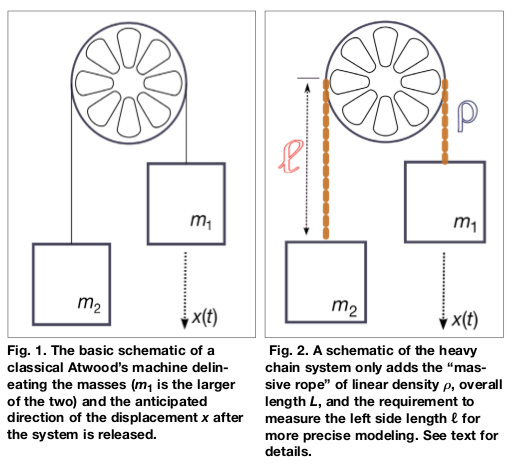This interesting problem was recently found in certain public examination. I will solve it here since I found it fascinating and useful, since it is a problem with “variable mass”.

A pulley with negligible mass is given (see the figures above). Its radius is R. We tie two masses,andto the extremes of the pulley with the aid of a string. The string has a linear mass density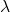and it has a total length equal to L. Calculate:

a) The forces in the extremes of the pulley over the masses and the acceleration when we release the system from the rest.

b) The velocity of the masses and the position of a the masses as a function of time.

Remark: We have the contraint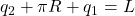. L is the total length of the string, R is the radius of the pulley, and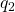and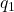are the pieces of string unwrapped to the pulley for the massesand, respectively. We can suppose that initially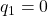and. Note that due to this constraint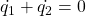, where the dot denotes derivative with respect to time, i.e., velocity of both masses, respecively too.

Solution.

a) Suppose. Thus, the forces acting on the masses are:Adding the two equations, we getand then the acceleration will beb) Firstly, we have to derive the differential equations for the system with variable mass.
The equations for both masses are, respectively, using Newton’s laws: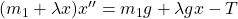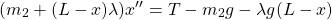Adding these two equations we get the differential equation to be solved: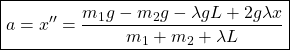withWe can solve the differential equationwith the initial conditions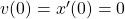and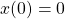easily, to obtainAs the mass of the string depends on the linear density, the usual procedure to obtain the variation of the kinetic energy as the work done by the potential forces is something more complicated:It can be shown thatPlease, note that in this case, we have to be very careful withand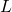. They are related with a contraint, but they are not the same thing.

This site uses Akismet to reduce spam. Learn how your comment data is processed.